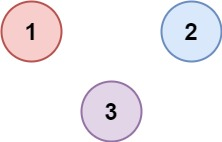547. Number of Provinces

There are `n` cities. Some of them are connected, while some are not. If city `a` is connected directly with city `b`, and city `b` is connected directly with city `c`, then city `a` is connected indirectly with city `c`.

A province is a group of directly or indirectly connected cities and no other cities outside of the group.

You are given an `n x n` matrix `isConnected` where `isConnected[i][j] = 1` if the `ith` city and the `jth` city are directly connected, and `isConnected[i][j] = 0` otherwise.

Return the total number of provinces.

Example 1:```Input: isConnected = [[1,1,0],[1,1,0],[0,0,1]]
Output: 2
```

Example 2:```Input: isConnected = [[1,0,0],[0,1,0],[0,0,1]]
Output: 3
```

Constraints:

• `1 <= n <= 200`
• `n == isConnected.length`
• `n == isConnected[i].length`
• `isConnected[i][j]` is `1` or `0`.
• `isConnected[i][i] == 1`
• `isConnected[i][j] == isConnected[j][i]`

547. Number of Provinces
``````struct Solution;

impl Solution {
fn dfs(m: &mut Vec<Vec<i32>>, visited: &mut Vec<bool>, i: usize, n: usize) {
for j in 0..n {
if m[i][j] == 1 && !visited[j] {
visited[j] = true;
Self::dfs(m, visited, j, n);
}
}
}

fn find_circle_num(mut m: Vec<Vec<i32>>) -> i32 {
let mut res = 0;
let n = m.len();
let mut visited: Vec<bool> = vec![false; n];
for i in 0..n {
if !visited[i] {
visited[i] = true;
Self::dfs(&mut m, &mut visited, i, n);
res += 1;
}
}
res
}
}

#[test]
fn test() {
let m: Vec<Vec<i32>> = vec_vec_i32![[1, 1, 0], [1, 1, 0], [0, 0, 1]];
assert_eq!(Solution::find_circle_num(m), 2);
let m: Vec<Vec<i32>> = vec_vec_i32![[1, 1, 0], [1, 1, 1], [0, 1, 1]];
assert_eq!(Solution::find_circle_num(m), 1);
}
``````